Miscellaneous

Chapter 8 Class 11 Sequences and Series
Serial order wise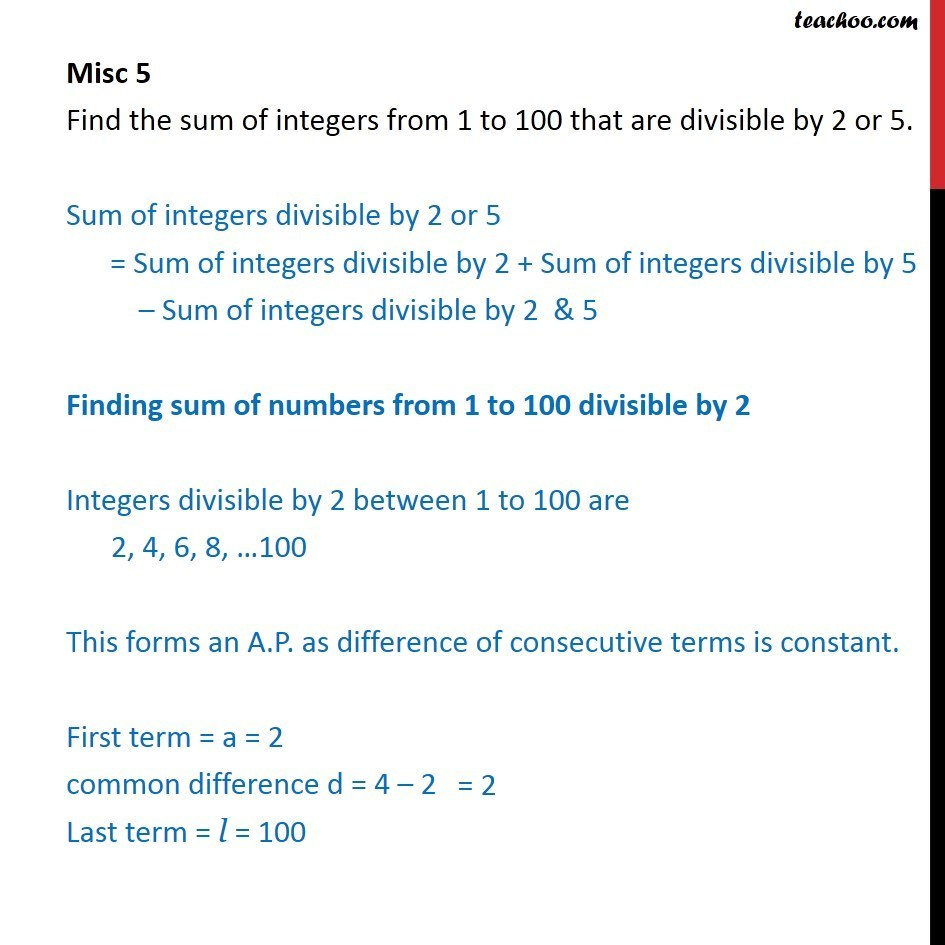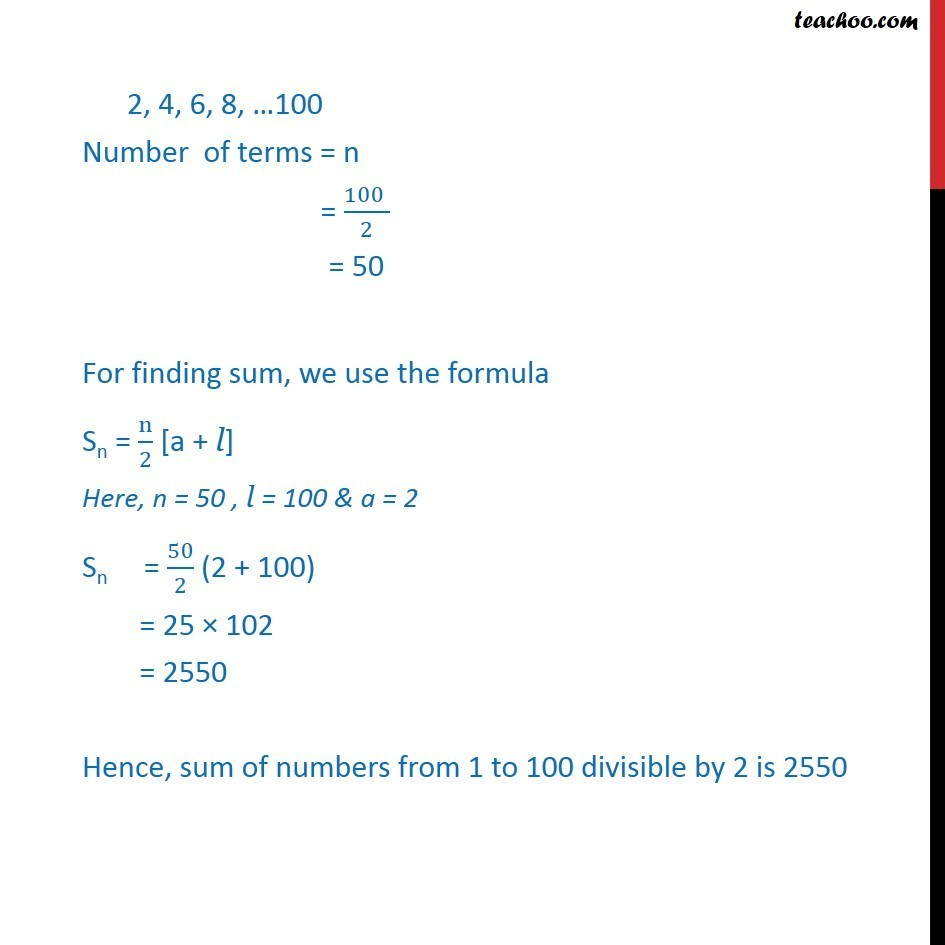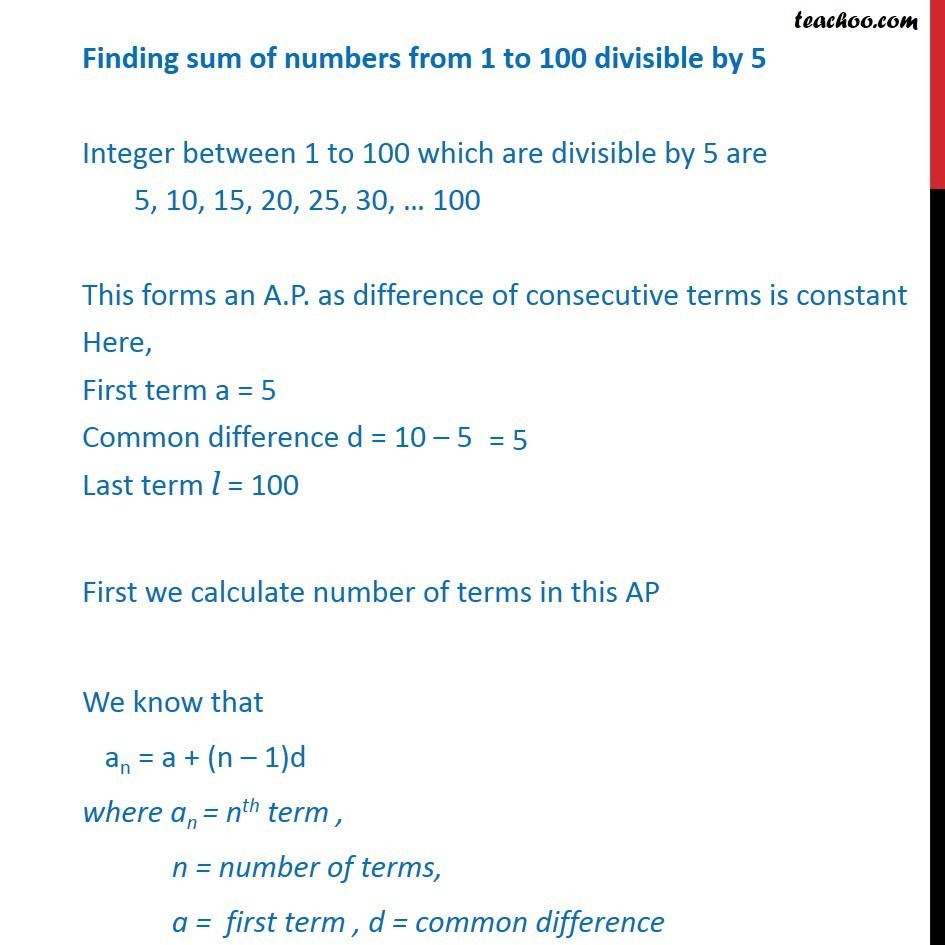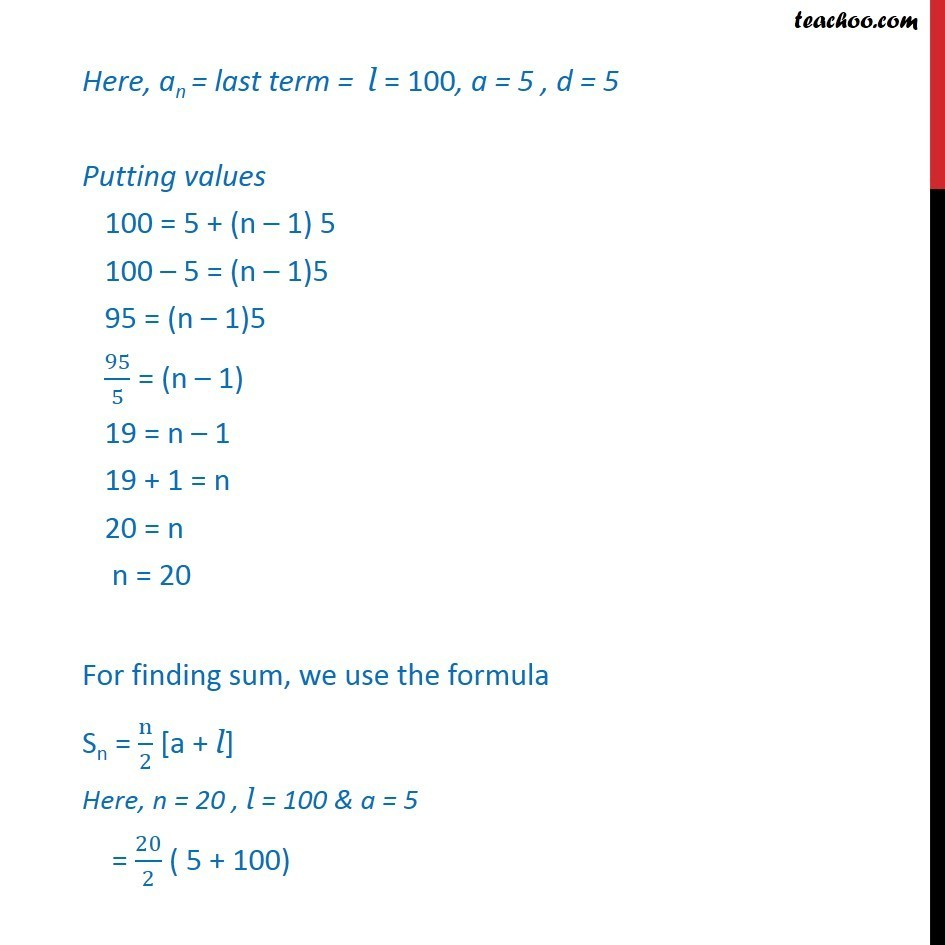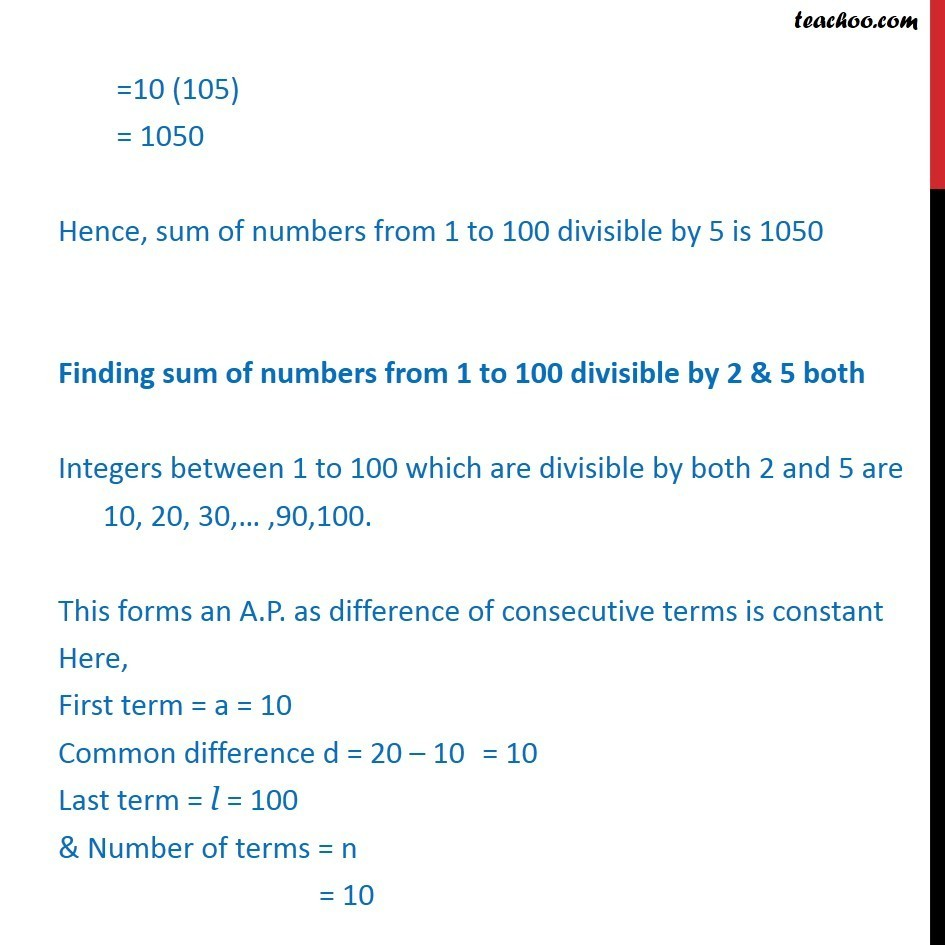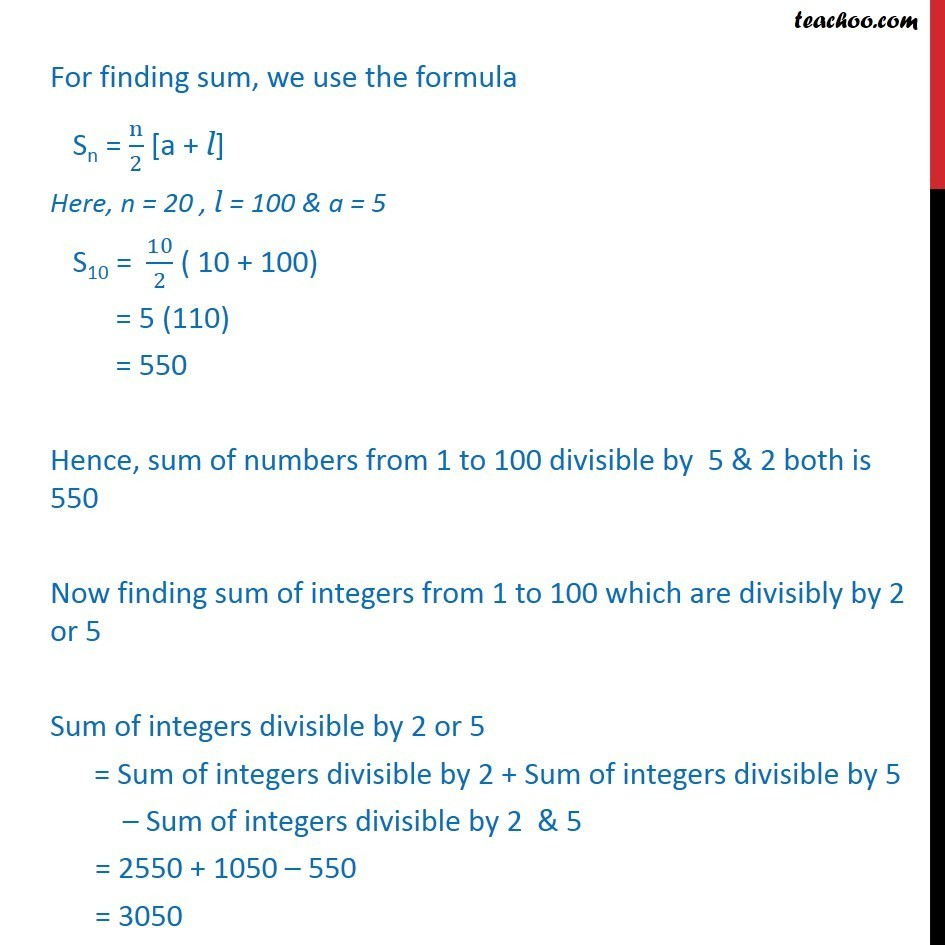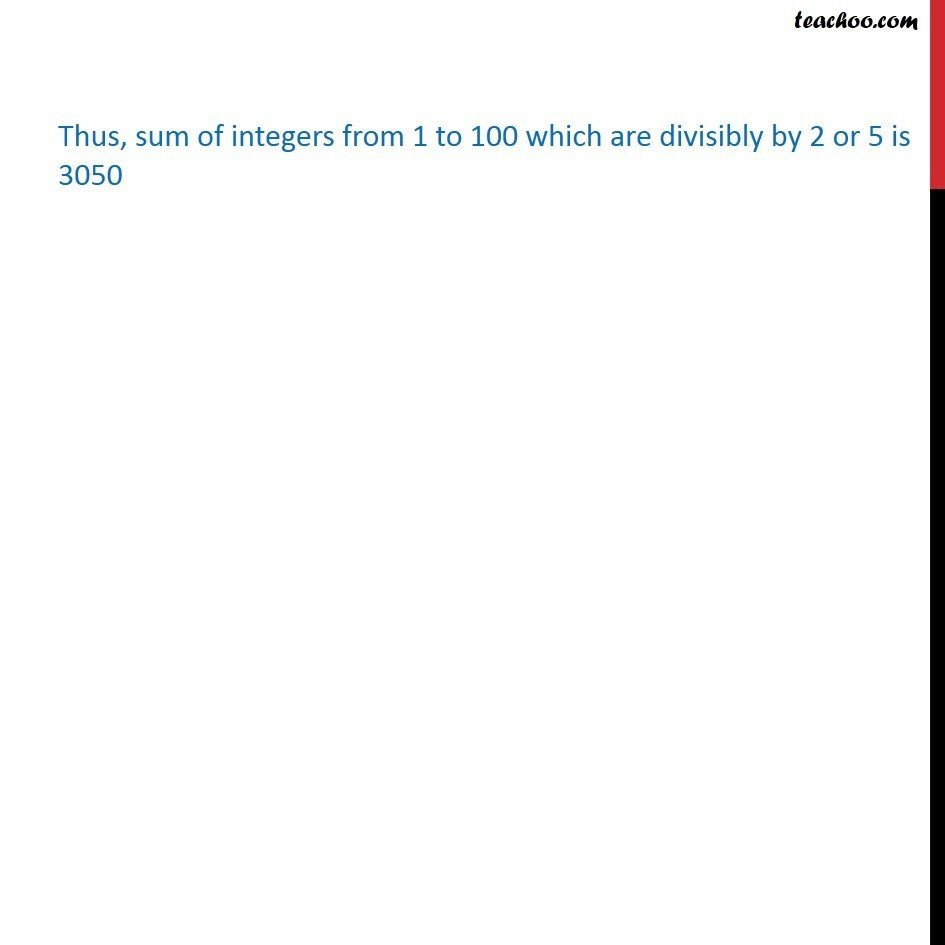Learn in your speed, with individual attention - Teachoo Maths 1-on-1 Class

### Transcript

Question 5 Find the sum of integers from 1 to 100 that are divisible by 2 or 5. Sum of integers divisible by 2 or 5 = Sum of integers divisible by 2 + Sum of integers divisible by 5 – Sum of integers divisible by 2 & 5 Finding sum of numbers from 1 to 100 divisible by 2 Integers divisible by 2 between 1 to 100 are 2, 4, 6, 8, …100 This forms an A.P. as difference of consecutive terms is constant. First term = a = 2 common difference d = 4 – 2 Last term = l = 100 2, 4, 6, 8, …100 Number of terms = n = (100 )/2 = 50 For finding sum, we use the formula Sn = n/2 [a + l] Here, n = 50 , l = 100 & a = 2 Sn = 50/2 (2 + 100) = 25 × 102 = 2550 Hence, sum of numbers from 1 to 100 divisible by 2 is 2550 Finding sum of numbers from 1 to 100 divisible by 5 Integer between 1 to 100 which are divisible by 5 are 5, 10, 15, 20, 25, 30, … 100 This forms an A.P. as difference of consecutive terms is constant Here, First term a = 5 Common difference d = 10 – 5 Last term l = 100 First we calculate number of terms in this AP We know that an = a + (n – 1)d where an = nth term , n = number of terms, a = first term , d = common difference Here, an = last term = l = 100, a = 5 , d = 5 Putting values 100 = 5 + (n – 1) 5 100 – 5 = (n – 1)5 95 = (n – 1)5 95/5 = (n – 1) 19 = n – 1 19 + 1 = n 20 = n n = 20 For finding sum, we use the formula Sn = n/2 [a + l] Here, n = 20 , l = 100 & a = 5 = 20/2 ( 5 + 100) =10 (105) = 1050 Hence, sum of numbers from 1 to 100 divisible by 5 is 1050 Finding sum of numbers from 1 to 100 divisible by 2 & 5 both Integers between 1 to 100 which are divisible by both 2 and 5 are 10, 20, 30,… ,90,100. This forms an A.P. as difference of consecutive terms is constant Here, First term = a = 10 Common difference d = 20 – 10 Last term = l = 100 & Number of terms = n = 10 For finding sum, we use the formula Sn = n/2 [a + l] Here, n = 20 , l = 100 & a = 5 S10 = 10/2 ( 10 + 100) = 5 (110) = 550 Hence, sum of numbers from 1 to 100 divisible by 5 & 2 both is 550 Now finding sum of integers from 1 to 100 which are divisibly by 2 or 5 Sum of integers divisible by 2 or 5 = Sum of integers divisible by 2 + Sum of integers divisible by 5 – Sum of integers divisible by 2 & 5 = 2550 + 1050 – 550 = 3050 Thus, sum of integers from 1 to 100 which are divisible by 2 or 5 is 3050